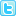ISSN: 0970-938X (Print) | 0976-1683 (Electronic)

# Biomedical Research

An International Journal of Medical Sciences

## Truncated logistic regression for matched case-control studies using data from vision screening for school children

Matching is a methodology applied at the beginning of a study that compares two or more groups. The main advantage for matching over random non-matching sampling is that matched study designs can often lead to a more statistically efficient analysis. The most commonly used methods to analyse the matched pair dataset are logistic regression models. The main advantage of using logistic regression with matched data occurs when there are variables other than the matched variables that the investigator wishes to control. Conditional logistic regression is one commonly used method to investigate the relationship between an outcome and a set of covariates in matched case-control studies. The aim of this study is to propose a truncated logistic regression analysis for matched case-control studies, an alternative approach to conditional logistic regression methods, and to demonstrate its applicability in matched case-control studies. We also applied the methods to a real data set and compared them. We showed that regression coefficient estimation can be accomplished using either truncated or conditional logistic regression methods. However, the parameter estimates for those covariates obtained from truncated logistic regression had more precision. In conclusion, truncated logistic regression model may be the preferred method for the analysis of matched case-control data because it is a more efficient method.

Author(s): Ertugrul Colak, Huseyin Gursoy
Abstract | Full-Text | PDF

Share this